求知 文章 文库 Lib 视频 iPerson 课程 认证 咨询 工具 讲座 Modeler Code分享到
HTML5高级编程之图形扭曲及其应用一

HTML5高级编程之图形扭曲及其应用一(原理篇)

HTML5高级编程之图形扭曲及其应用二(应用篇)

HTML5高级编程之图形扭曲及其应用一(原理篇)

HTML5中的几种变形

HTML5中的变形，共有以下几种方法

• scale() 缩放
• rotate() 旋转
• translate() 平移
• transform() 矩阵变形
• setTransform() 重设矩阵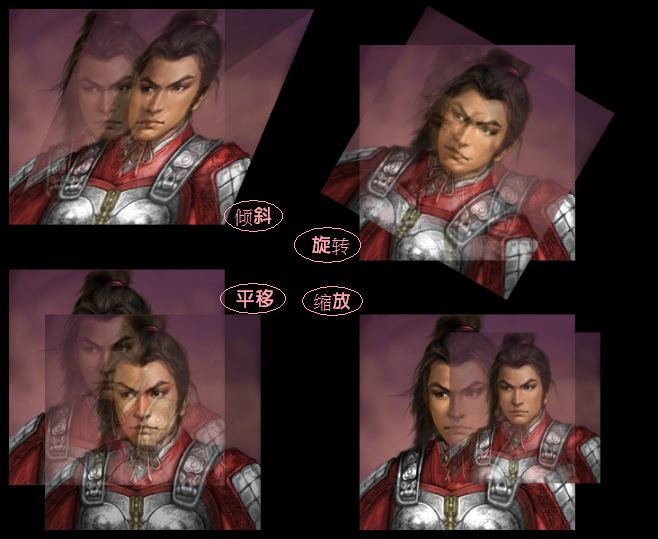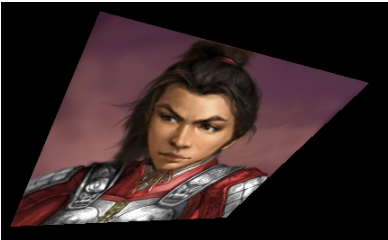1，缩放方法如下

 ``` ```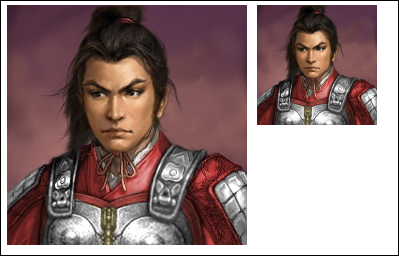2，旋转代码

 ``` ```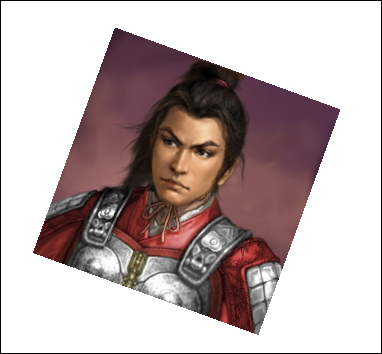3，平移代码

 ``` ```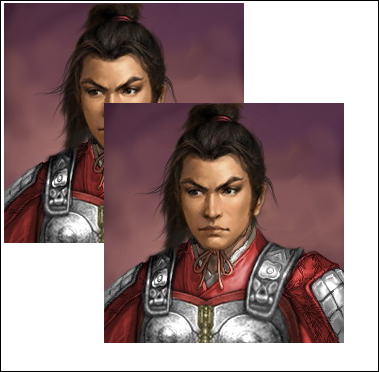4，倾斜代码

 ``` ```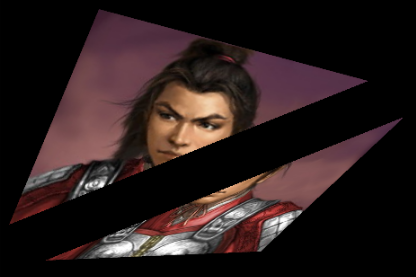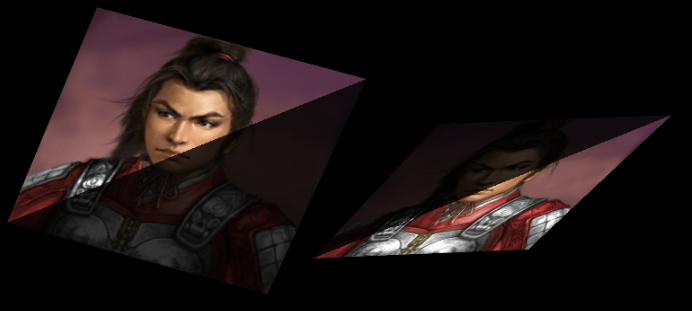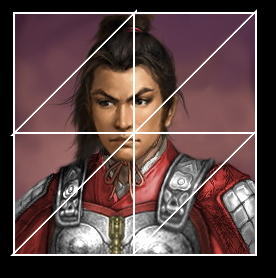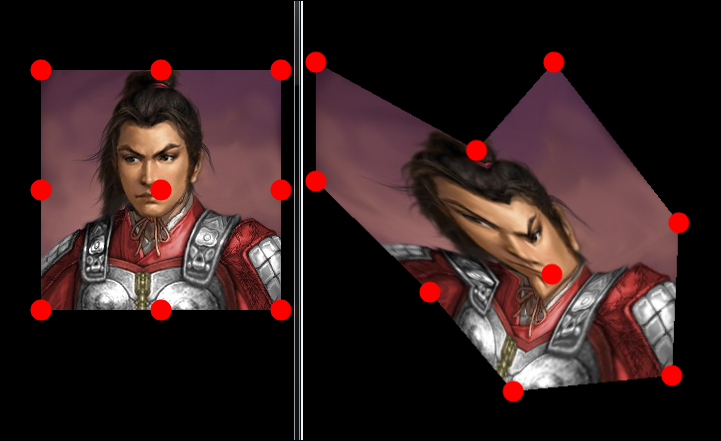http://lufy.netne.net/lufylegend-js/lufylegend-1.4/beginBitmapFill/sample01.html

HTML5高级编程之图形扭曲及其应用二(应用篇)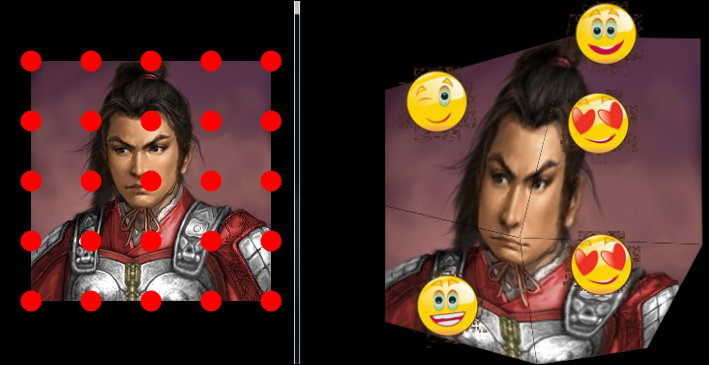drawTriangles函数的定义

 ```drawTriangles(vertices, indices, uvtData,thickness,color) vertices:由数字构成的矢量，其中的每一对数字将被视为一个坐标位置（一个 x, y 对）。vertices 参数是必需的。 indices:一个由整数或索引构成的矢量，其中每三个索引定义一个三角形。如果 indexes 参数为 null，则每三个顶点 （vertices 矢量中的 6 对 x,y）定义一个三角形。否则，每个索引将引用一个顶点，即 vertices 矢量中的一对数字。 例如，indexes 引用 (vertices, vertices)。 uvtData:由用于应用纹理映射的标准坐标构成的矢量。每个坐标引用用于填充的位图上的一个点。每个顶点必须具有一个UV 或 一个 UVT 坐标。对于 UV 坐标，(0,0) 是位图的左上角，(1,1) 是位图的右下角。 thickness:分割三角形的边框的线粗 color:分割三角形的边框的颜色```1，第一个参数vertices，其实就是定义每个顶点的坐标，这几个顶点的顺序依次为下图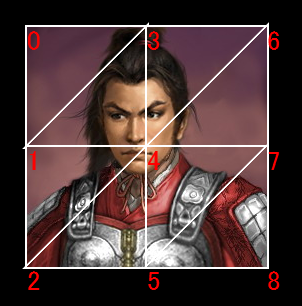vertices参数中储存的就是上面的9个顶点的坐标，代码如下

 ```vertices = new Array(); vertices.push(0, 0); vertices.push(0, 120); vertices.push(0, 240); vertices.push(120, 0); vertices.push(120, 120); vertices.push(120, 240); vertices.push(240, 0); vertices.push(240, 120); vertices.push(240, 240); ```

2，第二个参数indices是定义三角形，数组vertices中每三个顶点可以组成一个三角形，indices就是来定义这些三角形，这些三角形的顶点顺序是有规定的，其实从前面的图中可以看到，每两个三角形是一个矩形，定义这些三角形的时候，要以这些矩形的四个顶点为基准，三角形的顶点顺序分别是（左上，右上，左下）和（右上，左下，右下），如下图所示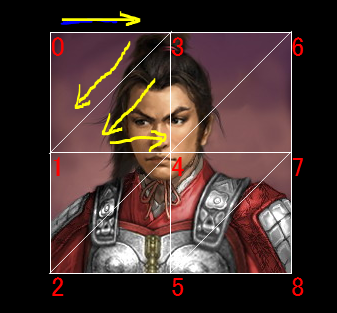```indices = new Array(); indices.push(0, 3, 1); indices.push(3, 1, 4); indices.push(1, 4, 2); indices.push(4, 2, 5); indices.push(3, 6, 4); indices.push(6, 4, 7); indices.push(4, 7, 5); indices.push(7, 5, 8); ```

2，第三个参数uvtData是定义上面的每个顶点相对于整张图片的比例，比如上面的图中的9个顶点的坐标，他们相对于原图片中的位置分别为下图所示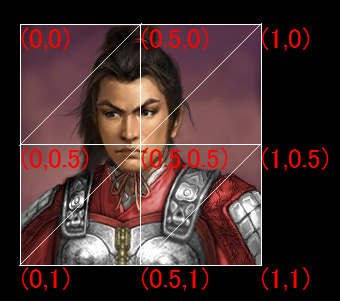```uvtData = new Array(); uvtData.push(0, 0); uvtData.push(0, 0.5); uvtData.push(0, 1); uvtData.push(0.5, 0); uvtData.push(0.5, 0.5); uvtData.push(0.5, 1); uvtData.push(1, 0); uvtData.push(1, 0.5); uvtData.push(1, 1); ```

beginBitmapFill是用位图图像填充绘图区，参数是LBitmapData对象

 ```vertices = new Array(); vertices.push(0, 0); vertices.push(0-50, 120);//这里将原坐标的x坐标左移50 vertices.push(0, 240); vertices.push(120, 0); vertices.push(120, 120); vertices.push(120, 240); vertices.push(240, 0); vertices.push(240+50, 120);//这里将原坐标的x坐标右移50 vertices.push(240, 240); indices = new Array(); indices.push(0, 3, 1); indices.push(3, 1, 4); indices.push(1, 4, 2); indices.push(4, 2, 5); indices.push(3, 6, 4); indices.push(6, 4, 7); indices.push(4, 7, 5); indices.push(7, 5, 8); uvtData = new Array(); uvtData.push(0, 0); uvtData.push(0, 0.5); uvtData.push(0, 1); uvtData.push(0.5, 0); uvtData.push(0.5, 0.5); uvtData.push(0.5, 1); uvtData.push(1, 0); uvtData.push(1, 0.5); uvtData.push(1, 1); backLayer.graphics.beginBitmapFill(bitmapData); backLayer.graphics.drawTriangles(vertices, indices, uvtData); ```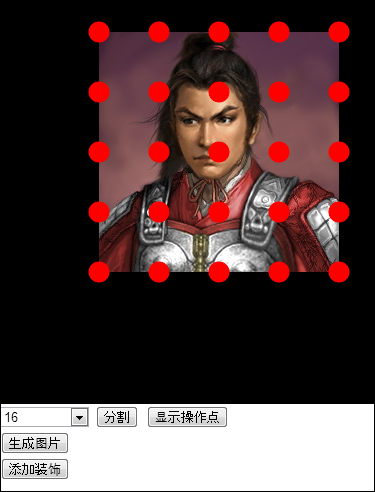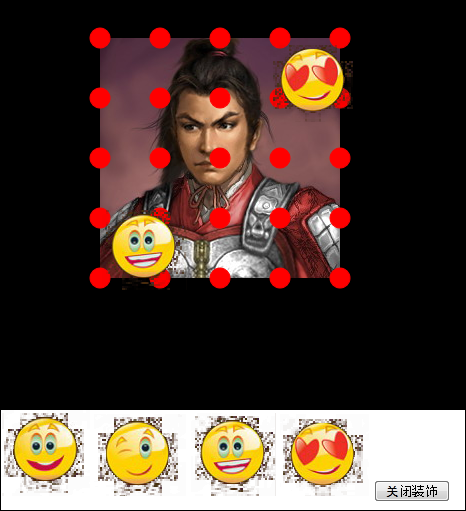http://lufylegend.com/html5/lufylegend/ps.html

HTML5高级编程之图形扭曲及其应用三(扩展篇)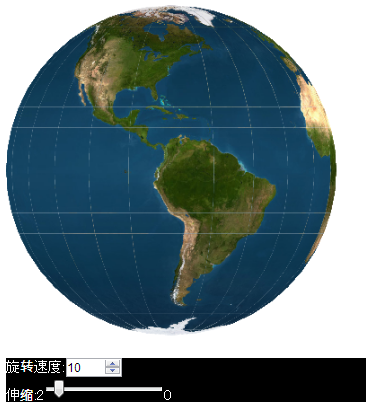http://lufylegend.com/lufylegend

 ```earthBitmapData = new LBitmapData("#ffffff", 0, 0, 500, 300); var i, j; vertices = new Array(); for(i=0;i<=cols;i++){ for(j=0;j<=rows;j++){ vertices.push(i*15,j*15); } } indices = new Array(); for (i = 0; i < cols; i++) { for (j = 0; j < rows; j++) { indices.push(i * (rows + 1) + j, (i + 1) * (rows + 1) + j, i * (rows + 1) + j + 1); indices.push((i + 1) * (rows + 1) + j, i * (rows + 1) + j + 1, (i + 1) * (rows + 1) + j + 1); } } uvtData = new Array(); for (i = 0; i <= cols; i++) { for (j = 0; j <= rows; j++) { uvtData.push(i / cols, j / rows); } }```

 ```backLayer = new LSprite(); addChild(backLayer); backLayer.graphics.clear(); backLayer.graphics.beginBitmapFill(earthBitmapData); backLayer.graphics.drawTriangles(vertices, indices, uvtData, 2);```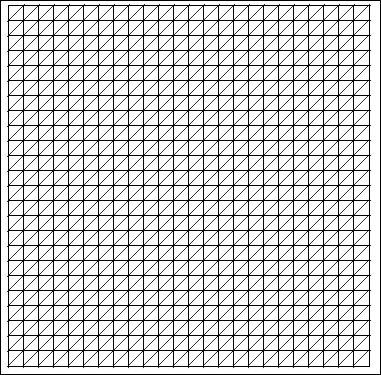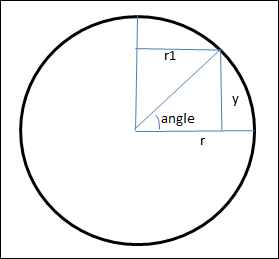```var a = Math.sin(angle); if((90-180*j/rows)%90==0 && (90-180*j/rows)%180!=0)a=(90-180*j/rows)>0?1:-1; var y = -r*a; var sa = Math.cos(angle); var r1 = Math.abs(r*sa); ```

 ```for(i=0;i<=cols;i++){ for(j=0;j<=rows;j++){ var angle = (90-180*j/rows)*Math.PI/180; var a = Math.sin(angle); if((90-180*j/rows)%90==0 && (90-180*j/rows)%180!=0)a=(90-180*j/rows)>0?1:-1; if((90-180*j/rows)%180==0)a=0; var sy = -r*a; vertices.push(i*15,sy); } } ```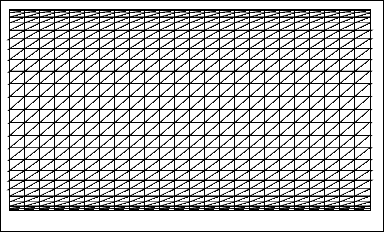```var b = Math.cos(angle*Math.PI/180); var x = r1*b; ```

 ```for(i=0;i<=cols;i++){ for(j=0;j<=rows;j++){ var sa = Math.cos(angle); if((90-180*j/rows)%180==0)sa=1; var sr = Math.abs(r*sa); var angle2 = 360*(i+1)/cols; var b = Math.cos(angle2*Math.PI/180); if(angle2%360==0)b=1; else if(angle2%180==0)b=-1; var sx = sr*b; vertices.push(sx,j*15); } } ```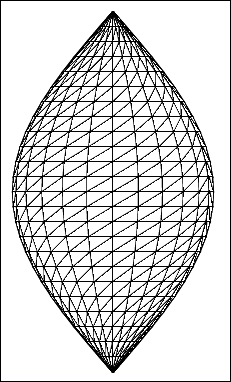```for(i=0;i<=cols;i++){ for(j=0;j<=rows;j++){ var angle = (90-180*j/rows)*Math.PI/180; var a = Math.sin(angle); if((90-180*j/rows)%90==0 && (90-180*j/rows)%180!=0)a=(90-180*j/rows)>0?1:-1; if((90-180*j/rows)%180==0)a=0; var sy = -r*a; var sa = Math.cos(angle); if((90-180*j/rows)%180==0)sa=1; var sr = Math.abs(r*sa); var angle2 = 360*(i+1)/cols; var b = Math.cos(angle2*Math.PI/180); if(angle2%360==0)b=1; else if(angle2%180==0)b=-1; var sx = sr*b; vertices.push(sx, sy); } } ```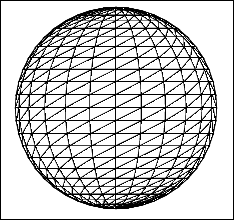`earthBitmapData = new LBitmapData(imglist["earth"]); `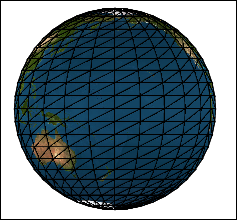```for (i = 0; i <= rows; i++) { uvtData.push(uvtData.shift()); uvtData.push(uvtData.shift()); } ```

http://lufy.netne.net/lufylegend-js/3dearth/index.html

http://lufylegend.com/lufylegend

 相关文章 深度解析：清理烂代码 如何编写出拥抱变化的代码 重构-使代码更简洁优美 团队项目开发"编码规范"系列文章
 相关文档 重构-改善既有代码的设计 软件重构v2 代码整洁之道 高质量编程规范
 相关课程 基于HTML5客户端、Web端的应用开发 HTML 5+CSS 开发 嵌入式C高质量编程 C++高级编程
 分享到

 十天学会DIV+CSS(WEB标准) HTML 5的革新：结构之美 介绍27款经典的CSS框架 35个有创意的404错误页面 最容易犯的13个JavaScript错误 设计易理解和操作的网站

 设计模式原理与应用 从需求过渡到设计 软件设计原理与实践 如何编写高质量代码 单元测试、重构及持续集成 软件开发过程指南

 东软集团 代码重构 某金融软件服务商 技术文档 中达电通 设计模式原理与实践 法国电信 技术文档编写与管理 西门子 嵌入式设计模式 中新大东方人寿 技术文档编写